# JAC Class 9 Maths Notes Chapter 5 Introduction to Euclid’s Geometry

Students should go through these JAC Class 9 Maths Notes Chapter 5 Introduction to Euclid’s Geometry will seemingly help to get a clear insight into all the important concepts.

### JAC Board Class 9 Maths Notes Chapter 5 Introduction to Euclid’s Geometry

Introduction:
The credit for introducing geometrical concepts goes to the distinguished Greek mathematician ‘Euclid’ who is known as the “Father of Geometry” and the word geometry comes from the Geek words ‘geo’ which means “Earth’ and ‘Metreon’ which means ‘measure’.

Basic Concepts In Geometry:
A point, a ‘line’ and a plane are the basic concepts to be used in geometry.
→ Axioms:
The statement that is taken to be true without proof, to serve as a premise for further reasoning and arguments, are called axioms.Euclid’s Definitions:

• A point is that which has no part.
• A line is breadthless length.
• The ends of a line segment are points.
• A straight line is that which has length only.
• A surface is that which has length and breadth only.
• The edges of surface are lines.
• A plane surface is a surface which lies evenly with the straight lines on itself.

Euclid’s Five Postulates:
→ A straight line may be drawn from any one point to any other point.
→ A terminated line or a line segment can be produced infinitely.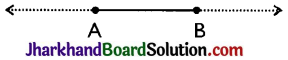→ A circle can be drawn with any centre and of any radius.
→ All right angles are equal to one another.
→ If a straight line falling on two straight lines makes the interior angles on the same side of it taken together less than two right angles, then the two straight lines, if produced infinitely, meet on that side on which the sum of angles is less than two right angles.

Important Axioms:
→ A line is the collection of infinite number of points.
→ Through a given point, infinite lines can be drawn.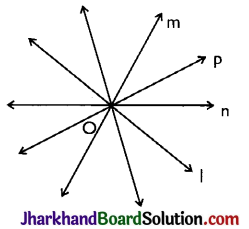→ Given two distinct points, there is one and only one line that contains both the points.
→ If P is a point not lying on a line l, then one and only one line can be drawn through P which is parallel to l.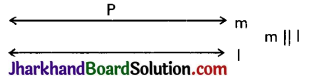→ Two distinct lines cannot have more than one point in common.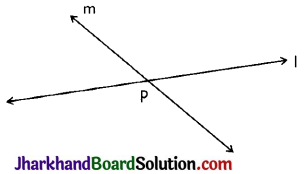→ Two lines which are both parallel to the same line, are parallel to each other. i.e. IF l || n, m || n ⇒ l || m.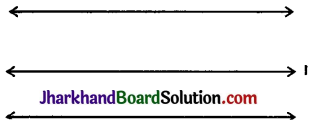Some Important Definitions:
→ Collinear points: Three or more points are said to be collinear if there is a line which contains all of them.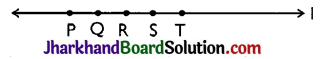→ Concurrent lines: Three or more lines are said to be concurrent if there is a point which lies on all of them.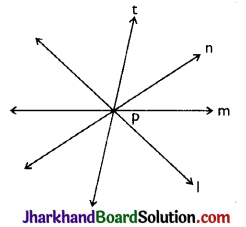→ Intersecting lines: Two lines are intersecting if they have a common point. The common point is called the “point of intersection”.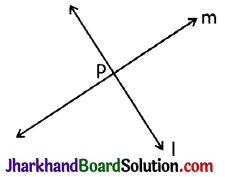→ Parallel lines: Two lines I and m in a plane are said to be parallel lines if they do not have a common point.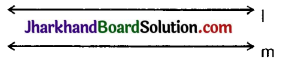→ Line segment: Given two points A and B on a line l, the connected part (segment) of the line with end points at A and B is called the line segment AB.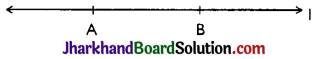→ Interior point of a line segment: A point R is called an interior point of a line segment PQ if R lies between Pand O but Ris neither P nor Q.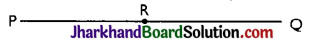→ Congruence of line segment: Two line segments AB and CD are congruent if trace copy of one can be superposed on the other so as to cover it completely and exactly in this case we write AB ≅ CD. In other words we can say two lines are congruent if their lengths are same.
→ Distance between two points: The distance between two points P and Q is the length of the line segment PO.
→ Ray: Directed line segment is called a ray. If AB is a ray, then it is denoted by $$\overrightarrow{\mathrm{AB}}$$. Point A is called initial point of ray.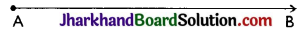→ Opposite rays: Two rays AB and AC are said to be opposite rays if they are collinear and point A is the only common point of the two rays and A lies in between B and C.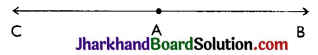Theorem 1.
If l, m, n are lines in the same plane such that l intersects m and n || m, then l also intersects n.
Given: Three lines l, m, n in the same plane such that intersects m and n || m.
To prove: Lines land n are intersecting lines.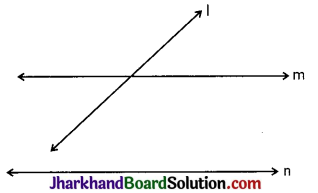Proof: Let l and n be non intersecting lines. Then. l || n. But, n || m [Given]
∴ l || n and n || m
⇒ l || m
⇒ l and m are non-intersecting lines.
This is a contradiction to the hypothesis that I and m are intersecting lines. So our supposition is wrong.
Hence, l intersects line n.Theorem 2.
If lines AB, AC, AD and AE are parallel to a line l, then points A, B, C, D and E are collinear.